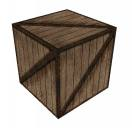Prev: Simple Texture Next: Multi-Texture

OpenGL allows us to combine the texture color with the fragments color in several ways. In the next table some of the available modes for the RGBA mode are presented:

 GL_REPLACE C = Ct A = At GL_MODULATE C = Ct*Cf A = At*Af GL_DECAL C = Cf * (1 – At) + Ct * At A = Af

In the table above Ct and At represent the color and alpha value of the texture element, Cf and Af represent the color and alpha value of the fragment (prior to applying the texture), and finally C and A represent the final color and alpha.

The example provided in the previous section is the equivalent of GL_REPLACE. Now we’re going to implement the equivalent of GL_MODULATE on a lit cube. The shaders will only compute the diffuse and ambient component with a white diffuse directional light. For the full material definition please see the lighting section.

Since we’re using lights, and therefore normals, the vertex shader must do some extra work. Namely it must transform into eye space and normalize the normal, and it must also normalize the light direction (the light direction has already been transformed into eye space by OpenGL). The vertex shader is now:

```varying vec3 lightDir,normal;

void main()
{
normal = normalize(gl_NormalMatrix * gl_Normal);

lightDir = normalize(vec3(gl_LightSource.position));
gl_TexCoord = gl_MultiTexCoord0;

gl_Position = ftransform();
}```

In the fragment shader the color and alpha of the lit fragment is computed into cf and af respectively. The rest of the shader is just computing the GL_MODULATE formulas presented above.

```varying vec3 lightDir,normal;
uniform sampler2D tex;

void main()
{
vec3 ct,cf;
vec4 texel;
float intensity,at,af;
intensity = max(dot(lightDir,normalize(normal)),0.0);

cf = intensity * (gl_FrontMaterial.diffuse).rgb +
gl_FrontMaterial.ambient.rgb;
af = gl_FrontMaterial.diffuse.a;
texel = texture2D(tex,gl_TexCoord.st);

ct = texel.rgb;
at = texel.a;
gl_FragColor = vec4(ct * cf, at * af);

}```GL_REPLACE GL_MODULATE

A Shader Designer project is available in here.

 Prev: Simple Texture Next: Multi-Texture

### 4 Responses to “Combine Texture + Fragment”

1.Hey,

nice tutorials but I have a question. I don’t undertand why normal will normalize in the Fragement shader again because it is normalize in the vertex shader or not?

•Hi,

That’s due to interpolation occuring between the vertex and fragment shader. The interpolation between two different normalised normals, in the general case, will result in an unnormalized vector.

See the pages on “interpolation issues” and “spaces and matrices” on the GLSL tutorial for more info.

2.Hi, Thanks for this tutorial. In my scene, I have objects with textures and others without. With this shader, only textured objects are rendered. I don’t understand how to improve this shader to do this.

•Hi Norris,

You either write two separate shaders. one for objects with textures and one for without, or you pass a flag stating whether the object uses textures. The flag can then be used to decide if you sample the texture or not.

Hope this helps,

Antonio

This site uses Akismet to reduce spam. Learn how your comment data is processed.